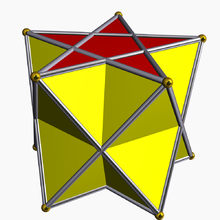# Prismatic uniform polyhedron

﻿
Prismatic uniform polyhedronA pentagrammic antiprism is made of two regular pentagrams and 10 equilateral triangles.

In geometry, a prismatic uniform polyhedron is a uniform polyhedron with dihedral symmetry. They exist in two infinite families, the uniform prisms and the uniform antiprisms. All have their vertices in parallel planes and are therefore prismatoids.

## Vertex configuration and symmetry groups

Because they are isogonal (vertex-transitive), their vertex arrangement uniquely corresponds to a symmetry group.

The difference between the prismatic and antiprismatic symmetry groups is that Dph has the vertices lined up in both planes, which gives it a reflection plane perpendicular to its p-fold axis (parallel to the {p/q} polygon); while Dpd has the vertices twisted relative to the other plane, which gives it a rotatory reflection. Each has p reflection planes which contain the p-fold axis.

The Dph symmetry group contains inversion if and only if p is even, while Dpd contains inversion symmetry if and only if p is odd.

## Enumeration

There are:

• prisms, for each rational number p/q > 2, with symmetry group Dph;
• antiprisms, for each rational number p/q > 3/2, with symmetry group Dpd if q is odd, Dph if q is even.

If p/q is an integer, i.e. if q = 1, the prism or antiprism is convex. (The fraction is always assumed to be stated in lowest terms.)

An antiprism with p/q < 2 is crossed or retrograde; its vertex figure resembles a bowtie. If p/q ≤ 3/2 no uniform antiprism can exist, as its vertex figure would have to violate the triangle inequality.

## Images

Note: The cube and octahedron are listed here with dihedral symmetry (as a square prism and triangular antiprism respectively), although if uniformly colored, they also have octahedral symmetry.

Wikimedia Foundation. 2010.

### Look at other dictionaries:

• Uniform polyhedron — A uniform polyhedron is a polyhedron which has regular polygons as faces and is transitive on its vertices (i.e. there is an isometry mapping any vertex onto any other). It follows that all vertices are congruent, and the polyhedron has a high… …   Wikipedia

• Uniform star polyhedron — A display of uniform polyhedra at the Science Museum in London …   Wikipedia

• Uniform polytope — A uniform polytope is a vertex transitive polytope made from uniform polytope facets. A uniform polytope must also have only regular polygon faces.Uniformity is a generalization of the older category semiregular, but also includes the regular… …   Wikipedia

• Polyhedron — Polyhedra redirects here. For the relational database system, see Polyhedra DBMS. For the game magazine, see Polyhedron (magazine). For the scientific journal, see Polyhedron (journal). Some Polyhedra Dodecahedron (Regular polyhedron) …   Wikipedia

• Prismatic compound of antiprisms with rotational freedom — Each member of this infinite family of uniform polyhedron compounds is a symmetric arrangement of antiprisms sharing a common axis of rotational symmetry. It arises from superimposing two copies of the corresponding prismatic compound of… …   Wikipedia

• Prismatic compound of prisms with rotational freedom — Each member of this infinite family of uniform polyhedron compounds is a symmetric arrangement of prisms sharing a common axis of rotational symmetry. It arises from superimposing two copies of the corresponding prismatic compound of prisms… …   Wikipedia

• Prismatic compound of prisms — Each member of this infinite family of uniform polyhedron compounds is a symmetric arrangement of prisms sharing a common axis of rotational symmetry.This infinite family can be enumerated as follows: *For each positive integer n >1 and for each… …   Wikipedia

• Prismatic compound of antiprisms — Each member of this infinite family of uniform polyhedron compounds is a symmetric arrangement of antiprisms sharing a common axis of rotational symmetry.This infinite family can be enumerated as follows: *For each positive integer n >1 and for… …   Wikipedia

• Uniform polychoron — In geometry, a uniform polychoron (plural: uniform polychora) is a polychoron or 4 polytope which is vertex transitive and whose cells are uniform polyhedra.This article contains the complete list of 64 non prismatic convex uniform polychora, and …   Wikipedia

• Convex uniform honeycomb — The alternated cubic honeycomb is one of 28 space filling uniform tessellations in Euclidean 3 space, composed of alternating yellow tetrahedra and red octahedra. In geometry, a convex uniform honeycomb is a uniform tessellation which fills three …   Wikipedia